Two workers

Two workers work together and perform the job in 12 hours. If one has done half the work and then second worker next half of work it will take 25 hours. How long would it take job them separately?

Result

a =  20 h
b =  30 h

Solution:Leave us a comment of this math problem and its solution (i.e. if it is still somewhat unclear...):Be the first to comment!To solve this verbal math problem are needed these knowledge from mathematics:

Looking for help with calculating harmonic mean? Looking for a statistical calculator? Do you have a system of equations and looking for calculator system of linear equations?

Next similar math problems:

1. Flowerbed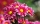Ondra digs the bed in 20 minutes. Cuba in 30 minutes. For how long do they dig together?
2. NovakNovak needed to dig up three of the same pit in the garden. The first pit dug father alone for 15 hours. His second dig son helped him and it did that in six hours. The third pit dug son himself. How long it took him?
3. Fire tank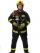Whole fire tank was discharged once in 5 days by first out by second in \$n days. Once firefighters pumped out 6/13 of water out from the tank and then let the water flow out both drain. How long take empty the tank?
4. Hectoliters of waterThe pool has a total of 126 hectoliters of water. The first pump draws 2.1 liters of water per second. A second pump pumps 3.5 liters of water per second. How long will it take both pumps to drain four-fifths of the water at the same time?
5. Two valves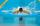Water fill the pool by two valves for 11 days. After 7 days the first valve was stopped and the second valve fill pool for 7 days. How many days took to fill the pool each of the valves individually?
6. Three workshopsThere are 2743 people working in three workshops. In the second workshop works 140 people more than in the first and in third works 4.2 times more than the second one. How many people work in each workshop?
7. Harmonic and arithmetic means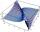The local Utah Department of Child Service office wants to project staffing needs based on current social worker assignments. They have the number of cases per social worker for the following staff: Mary: 25 John: 35 Ted: 15 Lisa: 45 Anna: 20 Calculat
8. FamilyFamily has 4 children. Ondra is 3 years older than Matthew and Karlos 5 years older than the youngest Jane. We know that they are together 30 years and 3 years ago they were together 19 years. Determine how old the children are.
9. Theorem proveWe want to prove the sentence: If the natural number n is divisible by six, then n is divisible by three. From what assumption we started?
10. Linsys2Solve two equations with two unknowns: 400x+120y=147.2 350x+200y=144
11. Lee isLee is 8 years more than twice Park's age, 4 years ago, Lee was three times as old. How old was Lee 4 years ago?
12. Father 7Father is 6 times older than his son. After 4 years, the father will only be 4 times older. What are their present ages?
13. PoojaPooja and Deepa age is 4:5, 4 years back it was 8:11. What is the age of Pooja now?
14. Inverse matrix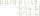Find out inverse by Gauss elimination or by reduction method. A=[2/3. 1 -3. 1/3]
15. Elimination methodSolve system of linear equations by elimination method: 5/2x + 3/5y= 4/15 1/2x + 2/5y= 2/15
16. Three unknownsSolve the system of linear equations with three unknowns: A + B + C = 14 B - A - C = 4 2A - B + C = 0
17. LegsCancer has 5 pairs of legs. The insect has 6 legs. 60 animals have a total of 500 legs. How much more are cancers than insects?